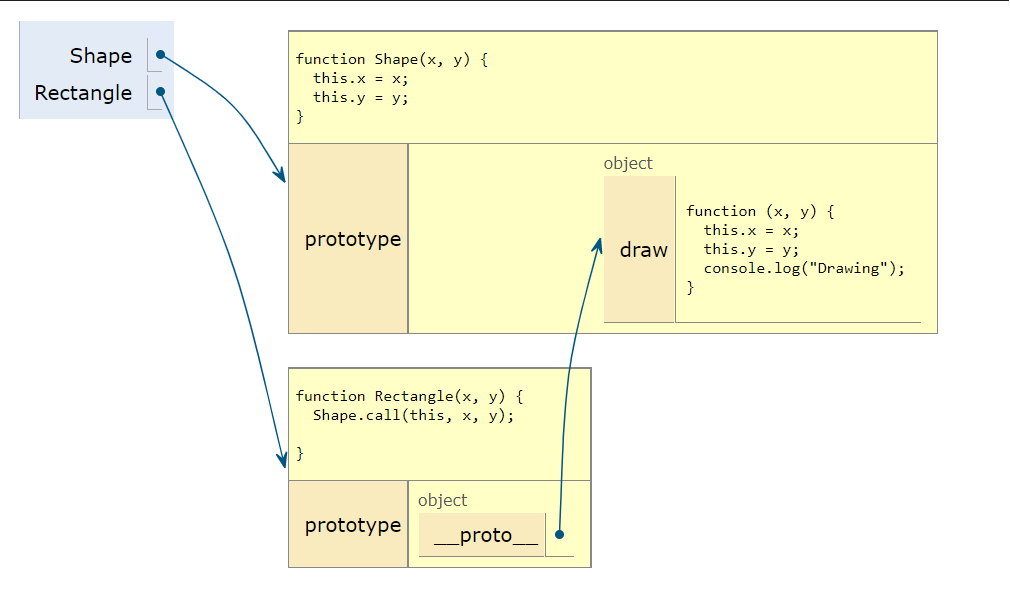# JavaScript Prototype Inheritance

In this article, I will show you how to implement inheritance in javascript. To understand the inheritance, let's get familiar with some terms.

### `__proto__` property

Every object in Javascript has its own secret, an internal attribute called `[[Prototype]]`. We can get to that `[[Prototype]]` by utilising the `__proto__` property. This instructs the computer to identify the prototype object as a hidden type. This prototype object must be connected to JavaScript objects. The inheritor object may now access an object’s properties.

### The Prototype Chain

Prototypal inheritance uses the concept of prototype chaining. Let’s explore that concept. Every object created contains `[[Prototype]]`, which points either to another object or null.

Consider the following example. In the following example, I have created two constructor function `Shape` and `Rectange`. Shape prototype has one function `draw`

``````
function Shape(x, y) {
this.x = x;
this.y = y;
}

Shape.prototype.draw = function(x, y) {
this.x = x;
this.y = y;
console.log("Drawing");
}

function Rectangle(x, y) {
Shape.call(this, x, y);

}
``````

To link the `Rectangle` prototype to `Shape` you can use `Object.create` the method from JavaScript. As you can see in the below image, that rectangle prototype is pointing to `Shape` prototype.

``````Rectangle.prototype = Object.create(Shape.prototype);
``````